Question

# Suppose an economy’s real GDP is \$50,000 in year 1 and \$51,200 in year 2. Instructions:...

Suppose an economy’s real GDP is \$50,000 in year 1 and \$51,200 in year 2. Instructions: Round your answers to 2 decimal places. a. What is the growth rate of its real GDP? percent. b. Assume that population is 100 in year 1 and 102 in year 2. What is the growth rate of real GDP per capita? percent.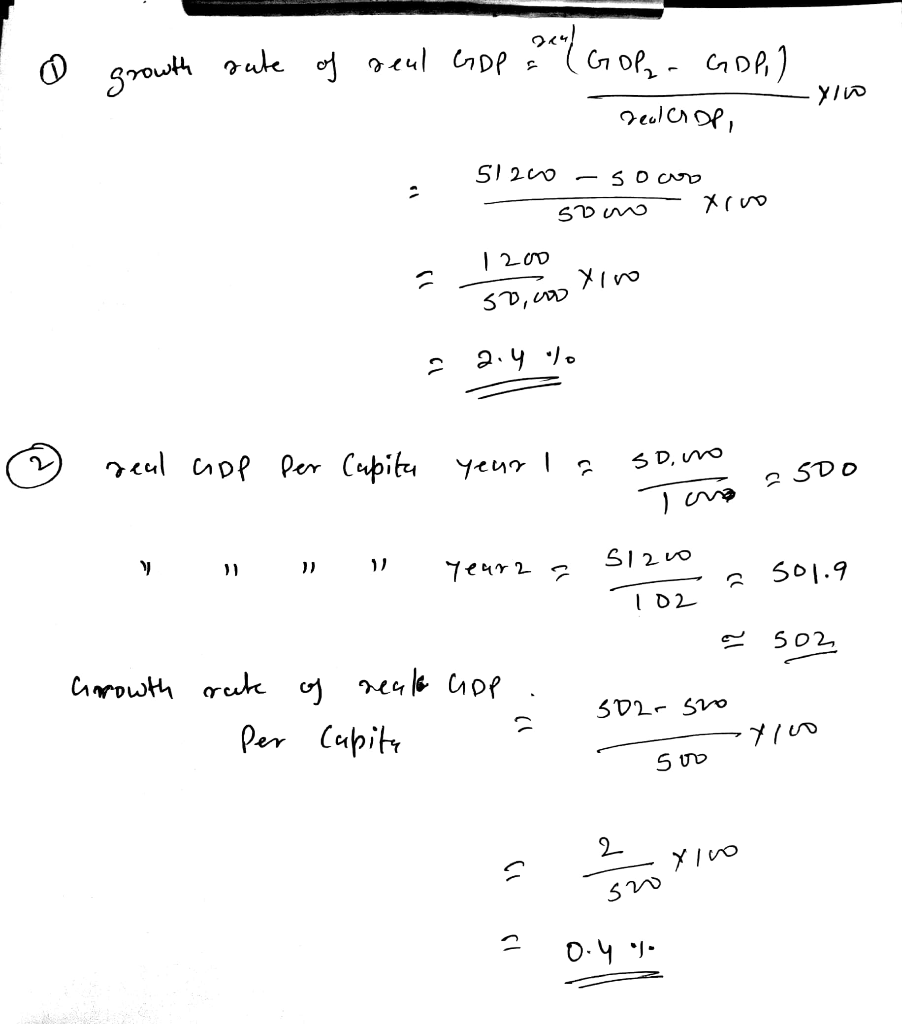#### Earn Coins

Coins can be redeemed for fabulous gifts.

Similar Homework Help Questions
• ### Suppose an economy's real GDP is \$30,000 in year 1 and \$31,200 in year 2. Instructions:...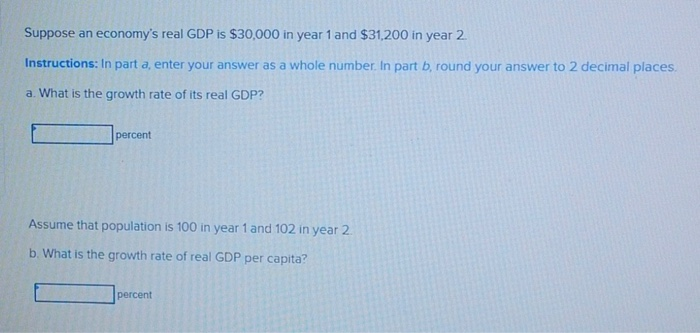Suppose an economy's real GDP is \$30,000 in year 1 and \$31,200 in year 2. Instructions: In part a, enter your answer as a whole number. In part b, round your answer to 2 decimal places a. What is the growth rate of its real GDP? percent Assume that population is 100 in year 1 and 102 in year 2. b. What is the growth rate of real GDP per capita? percent

• ### Reference equation: Real GDP per capita growth rate = Nominal GDP per capita growth rate -...

Reference equation: Real GDP per capita growth rate = Nominal GDP per capita growth rate - Inflation rate - Population growth rateThis equation is an approximation of the exact rate of growth of GDP per capita, and so it results in some errors when calculating this rate. However, the simplified equation both is easy to use and results in small error terms when inflation, nominal GDP growth, and population growth are low, and so it is a useful approximation. The...

• ### Reference equation: Real GDP per capita growth rate = Nominal GDP per capita growth rate-inflation rate-Population...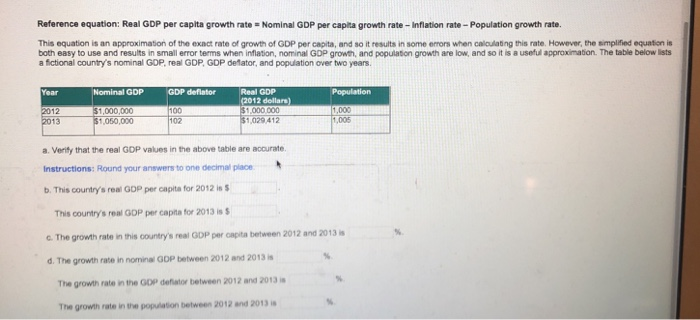Reference equation: Real GDP per capita growth rate = Nominal GDP per capita growth rate-inflation rate-Population growth rate This equation is an approximation of the exact rate of growth of GDP per capita, and so it results in some errors when caloulating this rate. However, the smplified equation is both easy to use and results in small error terms when inflation, nominal GDP growth, and population growth are low, and so it is a useful approximation. The table below lists...

• ### Reference equation: Real GDP per capita growth rate Nominal GDP per capita growth rate - Inflation...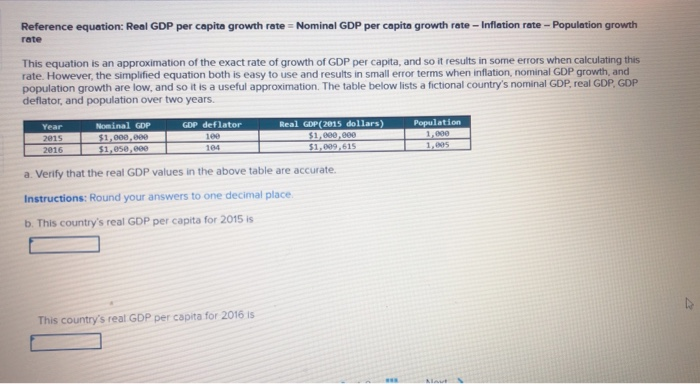Reference equation: Real GDP per capita growth rate Nominal GDP per capita growth rate - Inflation rate - Population growth rate This equation is an approximation of the exact rate of growth of GDP per capita, and so it results in some errors when calculating this rate. However, the simplified equation both is easy to use and results in small error terms when inflation, nominal GDP growth, and population growth are low, and so it is a useful approximation. The...

• ### Suppose a country wanted to increase the rate of growth of its per capita real GDP....

Suppose a country wanted to increase the rate of growth of its per capita real GDP. It could do this by A.decreasing the growth rate of real GDP and decreasing the population growth rate. B.decreasing the growth rate of real GDP and increasing the population growth rate. C.increasing the growth rate of real GDP and increasing the population growth rate. D.increasing the growth rate of real GDP and decreasing the population growth rate.

• ### Country A starts with real GDP per capita equal to \$ 40,000 and Country B starts with real GDP per capita equal to \$ 2,000 .

Country A starts with real GDP per capita equal to \$ 40,000 and Country B starts with real GDP per capita equal to \$ 2,000 .Today the RGDP per capita in A is _______ times the value in B.Country A is growing at a rate of 3.5 % per year and Country B is growing at a rate of 7 % per year. Assume these growth rates do not change.Country A will double its RGDP per capita in _______ years...

• ### Suppose a country's real GDP is \$14 trillion and that population is 200 million

Suppose a country's real GDP is \$14 trillion and that population is 200 million Instructions: Enter your answers as whole numbers a. What is this country's real GDP per capita? Suppose that during the next 10 years, real GDP grows by half and population triples in the country. b. After 10 years have passed, what will be this country's real GDP per capita?

• ### Suppose a country's real GDP is \$18 trillion and that population is 300 million.

Suppose a country's real GDP is \$18 trillion and that population is 300 million. Instructions: Enter your answers as whole numbers. a. What is this country's real GDP per capita? Suppose that during the next 10 years, real GDP grows by half and population triples in the country. b. After 10 years have passed, what will be this country's real GDP per capita?

• ### The following table gives some figures from forecasts of real GDP in 2005 dollars) and population completed in mid-2014

The following table gives some figures from forecasts of real GDP in 2005 dollars) and population completed in mid-2014. Real GDP 2018   \$18, 121.00 billionReal GDP 2019   \$18,664.63 billionPopulation 2018  329.00 millionPopulation 2019  332.29 million The real GDP growth rate between 2018 and 2019 will be (3.00) %. (Round your response to two decimal places.) The population growth rate between 2018 and 2019 will be (1) %. (Round your response to two decimal places.) Per capita GDP in 2018 is projected to be \$ ....

• ### Q1) Q2) Q3) Imagine that Canada produces only three goods: apples, bananas, and carrots. The quantities...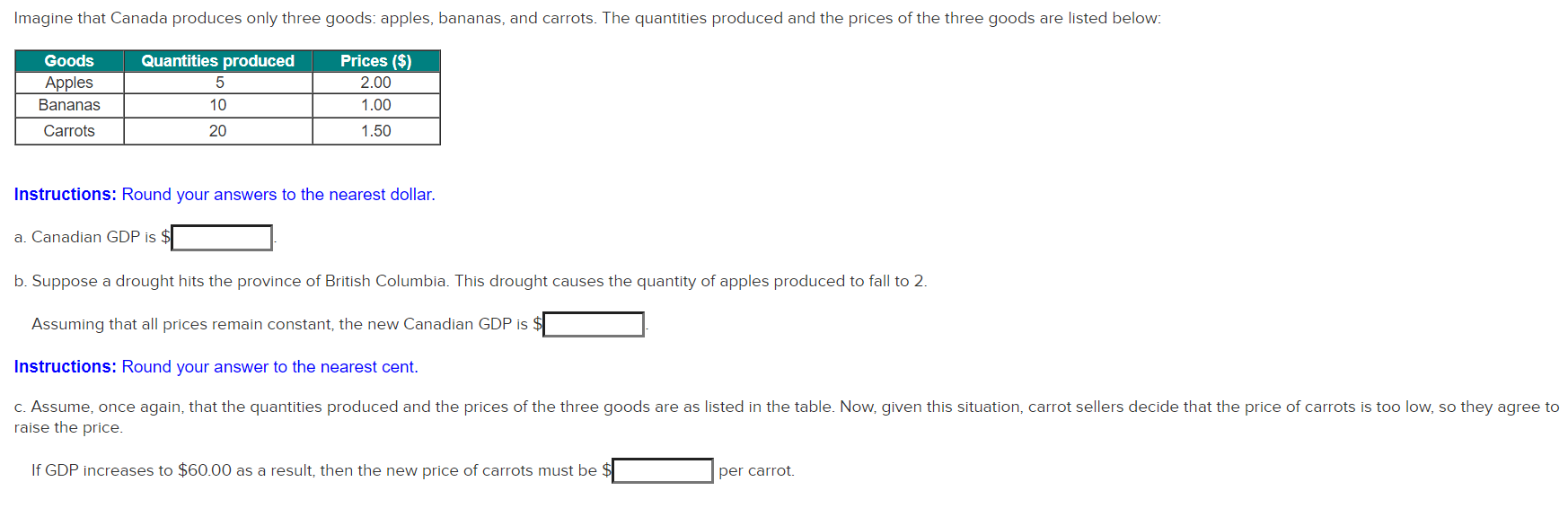Q1) Q2) Q3) Imagine that Canada produces only three goods: apples, bananas, and carrots. The quantities produced and the prices of the three goods are listed below: Quantities produced 5 Goods Apples Bananas Carrots Prices (\$) 2.00 1.00 10 20 1.50 Instructions: Round your answers to the nearest dollar. a. Canadian GDP is \$ b. Suppose a drought hits the province of British Columbia. This drought causes the quantity of apples produced to fall to 2. Assuming that all prices...